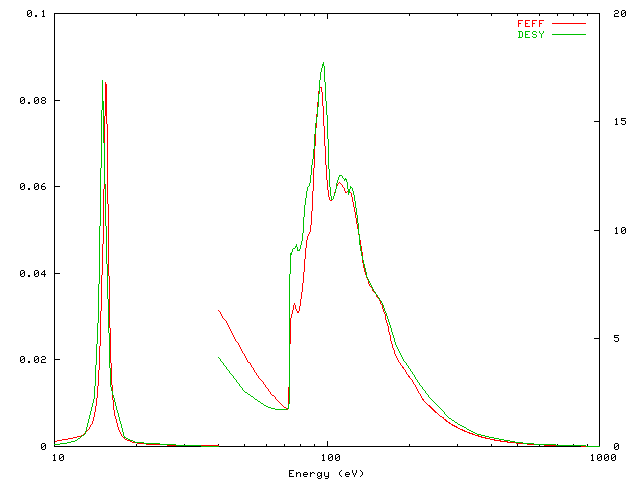## ab initio Optical Constants: UV through X-ray

Micah Prange
University of Washington

Objective: Create an automated code to calculate optical constants over the entire spectrum using the RSMS Green's function approach and test the method against experimental data. Employ our optical constants in applications.

• Frequency dependent optical properties. There is no comprehensive source for optical constants of arbitrary materials at arbitrary frequencies, even though optical constants have many applications. We have developed an extension of the x-ray spectroscopy code FEFF that calculates several optical constants in the energy range 0-100 keV. The code is based on a RSMS Green's function formalism that includes self-consistent scattering potentials, finite core-hole lifetimes, and photoelectron self-energy effects. We use a combination of a high order multiple scattering path expansion and a full multiple scattering matrix inversion to accurately compute the single particle photoelectron Green's function over a wide energy range. Our calculation proceeds by computing the imaginary part of the frequency-dependent dielectric constant by simulating all relavent absorption processes. Since all absorption processes have been simulated, we can perform a Kramers-Kronig analysis to calculate the real part of the dielectric constant. The index of refraction, normal incidence reflectivity, absorption coefficient, and energy loss spectrum are then computed from the dielectric function.

• Tests of Theory . As a check on the spectra, our code calculates several sum rules. As a further check, we have compared our results to tabulated optical constants produced by measurment for Mg, Al, Cu, Ag, and Au. We find that our present implementation reproduces experiemntal spectra above ~20 eV, and comment on refinements that might improve the low-energy portions of our spectra.

• Applications . We have used our calculated dielelectric functions to replace the the standard plasmon-pole self energy model used in the calculation of x-ray absorption spectra with an ab-initio multi-pole model, improving agreement with experiment. One can also calculate the inelastic mean free path for electrons from our results. We have done this and present a comparision wiht the standard Penn model. Finally, we present Hamaker coefficients calculated from our frequency dependent dielectric function.

Contact: Micah Prange, voice: 206-271-2646; prange@phys.washington.eduThe energy loss function in Al. The red curve is our calculation; the green curve is experimental.